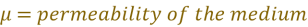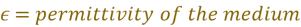General formulae for calculating wave velocity in different mediaGeneral formulae for calculating wave velocity in different media

1. Velocity of longitudinal wave in liquid and gases is given by: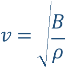Where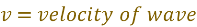,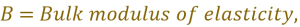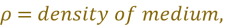1. Velocity of longitudinal waves in solid rod is given by: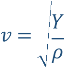Where,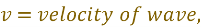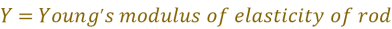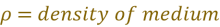1. Velocity of transverse wave on a stretched string is given by: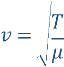Where,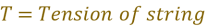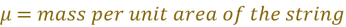1. Velocity of electromagnetic waves is given by: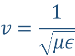Where,